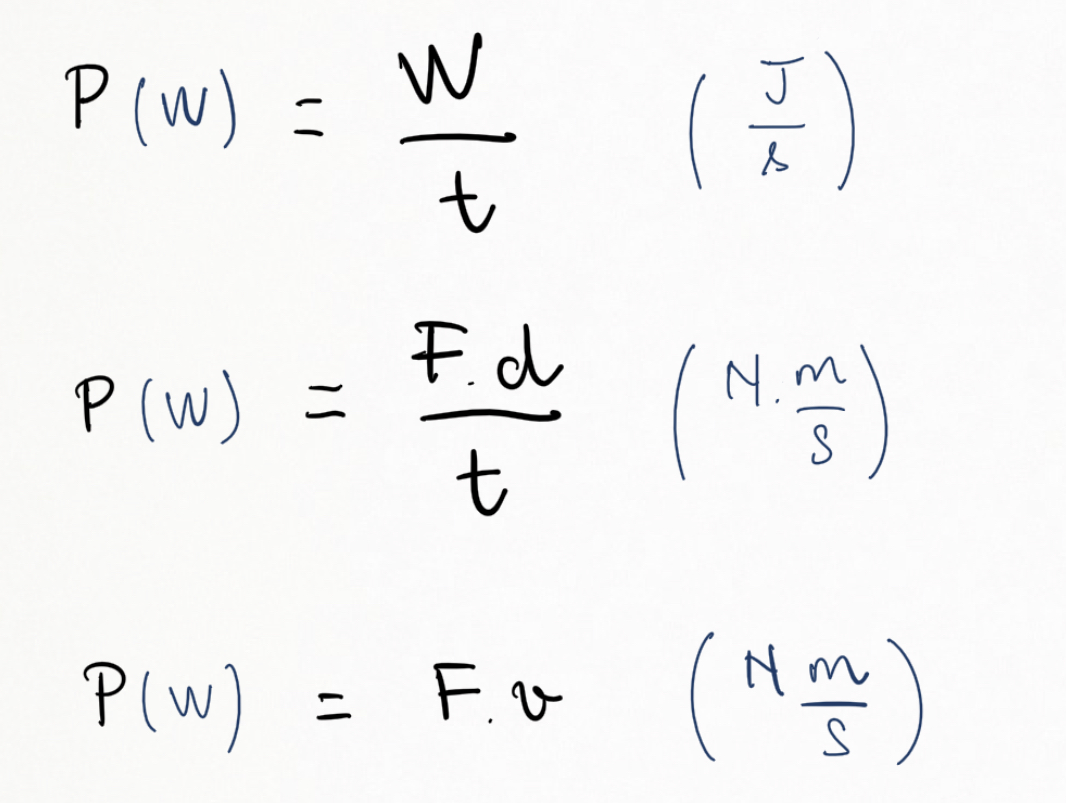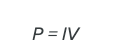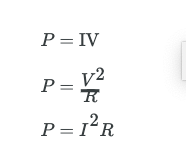### Power, units

Topic: Energy Of Point Object Systems

Power is the amount of energy produced per unit time. Power = Work/Time. This is equivalent to Force x Velocity.The unit of Power is Watt (W) which can also be measured as joules per second or Nm per second.

Power is also associated with electricity. Electric energy depends on both the voltage involved and the charge moved. This is expressed most simply as PE = qV, where q is the charge moved and V is the voltage (or more precisely, the potential difference the charge moves through). Power is the rate at which energy is moved, and so electric power isElectric power (P) is simply the product of current times voltage. Power has familiar units of watts. Since the SI unit for potential energy (PE) is the joule, power has units of joules per second, or watts. Thus, 1 A ⋅V= 1 W. For example, cars often have one or more auxiliary power outlets with which you can charge a cell phone or other electronic devices. These outlets may be rated at 20 A, so that the circuit can deliver a maximum power P = IV = (20 A)(12 V) = 240 W. In some applications, electric power may be expressed as volt-amperes or even kilovolt-amperes (1 kA ⋅V = 1 kW).

To see the relationship of power to resistance, we combine Ohm’s law with P = IV. Substituting I = V/R gives = (V/R)V=V2/R. Similarly, substituting V = IR gives P = I(IR) = I2R. Three expressions for electric power are listed together here for convenience:Practice Questions

MCAT Official Prep (AAMC)

Physics Online Flashcards Question 13

Physics Online Flashcards Question 22

Official Guide C/P Section Passage 2 Question 8

Practice Exam 1 C/P Section Question 59

Key Points

• Power is Work/Time or Force*speed

• The unit of Power is Watt (W)

• Power can be calculated by multiplying the voltage by the current in an electrical circuit

Key Terms

Power: The amount of energy produced per unit time

Watt: In the International System of Units, the derived unit of power; the power of a system in which one joule of energy is transferred per second.

Billing Information
We had trouble validating your card. It's possible your card provider is preventing us from charging the card. Please contact your card provider or customer support.
{{ cardForm.errors.get('number') }}
{{ registerForm.errors.get('zip') }}
{{ registerForm.errors.get('coupon') }}
Tax: {{ taxAmount(selectedPlan) | currency spark.currencySymbol }}

Total Price Including Tax: {{ priceWithTax(selectedPlan) | currency spark.currencySymbol }} / {{ selectedPlan.interval | capitalize }}